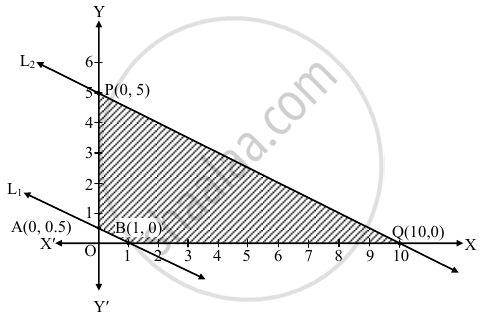# Choose the correct alternative : Solution of LPP to minimize z = 2x + 3y st. x ≥ 0, y ≥ 0, 1≤ x + 2y ≤ 10 is - Mathematics and Statistics

MCQ

Choose the correct alternative :

Solution of LPP to minimize z = 2x + 3y st. x ≥ 0, y ≥ 0, 1≤ x + 2y ≤ 10 is

#### Options

• x = 0, y = (1)/(2)

• x = (1)/(2), y = 0

• x = 1, y = – 2

• x = y = (1)/(2)

#### Solution

Z = 2x + 3y
The given inequalities are 1 ≤ x + 2y ≤ 10
i.e. x + 2y ≥ 1 and x + 2y ≤ 10
consider lines L1 and L2 where L1 : x + 2y = 1, L2 : x + 2y = 10.
For line L1 plot A(0, 1/2), B(1, 0)
For line L2 plot P (0, 5), Q (10, 0).
The coordinates of origin O (0, 0) do not satisfy x + 2y ≥ 1.
Required region lies on non – origin side of L1.
The coordinates of origin O(0, 0) satisfies the inequalities x + 2y ≤ 10.
Required region lies on the origin side of L2.
Lines L1 and L2 are parallel.
ABQPA is the required feasible region
At "A"(0, 1/2), "Z" =  0+ 3(1/2) = 1.5
At B (1, 0), Z = 2 (1) + 0 = 2
At P (0, 5), Z = 0 + 3(5) = 15
At Q (10, 0), Z = 2 (10) + 0 = 20
The maximum value of Z is 1.5 and it occurs at "A"(0, 1/2) i.e. x = 0, y = (1)/(2)Concept: Linear Programming Problem (L.P.P.)
Is there an error in this question or solution?
Chapter 6: Linear Programming - Miscellaneous Exercise 6 [Page 103]

#### APPEARS IN

Balbharati Mathematics and Statistics 2 (Commerce) 12th Standard HSC Maharashtra State Board
Chapter 6 Linear Programming
Miscellaneous Exercise 6 | Q 1.09 | Page 103
Share